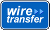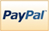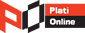Categories

View all categories

Fit curves and surfaces to data using regression, interpolation, and smoothing

App and functions for fitting curves and surfaces to data. The toolbox lets you perform exploratory data analysis, preprocess and post-process data, compare candidate models, and remove outliers. You can conduct regression analysis using the library of linear and nonlinear models provided or specify your own custom equations.  The library provides optimized solver parameters and starting conditions to improve the quality of your fits. The toolbox also supports nonparametric modeling techniques, such as splines, interpolation, and smoothing.

After creating a fit, you can apply a variety of post-processing methods for plotting, interpolation, and extrapolation; estimating confidence intervals; and calculating integrals and derivatives.

 Full Product 1.200,00 EUR
MTHWRKS-CUFITO
Math

Solve multiple maxima, multiple minima, and nonsmooth optimization problems

Provides methods that search for global solutions to problems that contain multiple maxima or minima. It includes global search, multistart, pattern search, genetic algorithm, and simulated annealing solvers. You can use these solvers to solve optimization problems where the objective or constraint function is continuous, discontinuous, stochastic, does not possess derivatives, or includes simulations or black-box functions with undefined values for some parameter settings.

Genetic algorithm and pattern search solvers support algorithmic customization. You can create a custom genetic algorithm variant by modifying initial population and fitness scaling options or by defining parent selection, crossover, and mutation functions. You can customize pattern search by defining polling, searching, and other functions.

 Full Product 1.200,00 EUR
MTHWRKS-GLOPTO
Math

Calibrate complex powertrain systems

Apps and design tools for optimally calibrating complex powertrain systems using statistical modeling and numeric optimization. You can define test plans, develop statistical models, and generate calibrations and lookup tables for complex high-degree-of-freedom engines that would require exhaustive testing using traditional methods. By using the toolbox with MATLAB and Simulink, you can develop a process for systematically identifying the optimal balance of engine performance, emissions, and fuel economy, and reuse statistical models for control design, hardware-in-the-loop testing, or powertrain simulation.

 Full Product 7.900,00 EUR
MTHWRKS-MOBACATO
Math

Create, train, and simulate neural networks

Functions and apps for modeling complex nonlinear systems that are not easily modeled with a closed-form equation. Neural Network Toolbox supports supervised learning with feedforward, radial basis, and dynamic networks. It also supports unsupervised learning with self-organizing maps and competitive layers. With the toolbox you can design, train, visualize, and simulate neural networks. You can use Neural Network Toolbox for applications such as data fitting, pattern recognition, clustering, time-series prediction, and dynamic system modeling and control.

To speed up training and handle large data sets, you can distribute computations and data across multicore processors, GPUs, and computer clusters using Parallel Computing Toolbox.

 Full Product 1.200,00 EUR
MTHWRKS-NENETO
Math

Algorithms for standard and large-scale optimization.

These algorithms solve constrained and unconstrained continuous and discrete problems. The toolbox’s optimization software includes functions for linear programming, quadratic programming, binary integer programming, nonlinear optimization, nonlinear least squares, systems of nonlinear equations, and multiobjective optimization. You can use them to find optimal solutions, perform tradeoff analyses, balance multiple design alternatives, and incorporate optimization methods into algorithms and models.

 Full Product 1.200,00 EUR
MTHWRKS-OPTO
Math

Solve partial differential equations using finite element methods

Contains tools for the study and solution of partial differential equations (PDEs) in two-space dimensions (2-D) and time. A PDE app and functions let you preprocess, solve, and postprocess generic 2-D PDEs for a broad range of engineering and science applications.

 Full Product 1.200,00 EUR
MTHWRKS-PSDIEQTO
Math

Analyze and model data using statistics and machine learning

Provides statistical and machine learning algorithms and tools for organizing, analyzing, and modeling data. You can use regression or classification for predictive modeling, generate random numbers for Monte Carlo simulations, use statistical plots for exploratory data analysis, and perform hypothesis tests.

For analyzing multidimensional data, Statistics Toolbox includes algorithms that let you identify key variables that impact your model with sequential feature selection, transform your data with principal component analysis, apply regularization and shrinkage, or use partial least squares regression. The toolbox provides supervised and unsupervised machine learning algorithms, including boosted and bagged decision trees, K-means and hierarchical clustering, K-nearest neighbor search, Gaussian mixtures, the expectation maximization algorithm, and hidden Markov models. With these algorithms you can extract meaning from your data and develop predictive models.

 Full Product 1.200,00 EUR
MTHWRKS-STTO
Math

Perform symbolic math computations

Provides functions for solving and manipulating symbolic math expressions and performing variable-precision arithmetic.

You can analytically perform differentiation, integration, simplification, transforms, and equation solving. You can also generate code for MATLAB, Simulink, and Simscape from symbolic math expressions.

Symbolic Math Toolbox includes the MuPAD language, which is optimized for handling and operating on symbolic math expressions. It provides libraries of MuPAD functions in common mathematical areas such as calculus and linear algebra and in specialized areas such as number theory and combinatorics. You can also write custom symbolic functions and libraries in the MuPAD language. The MuPAD Notebook app lets you document symbolic math derivations with embedded text, graphics, and typeset math. You can share the annotated derivations as HTML or as a PDF.

 Full Product 1.200,00 EUR
MTHWRKS-SYMATO
Math

 General Terms | Privacy Terms | Sales Terms | Become an Affiliate | Become a Reseller | Sitemap | Country:United States Language:1996 - 2019 © STIRA Electronic SRL Login